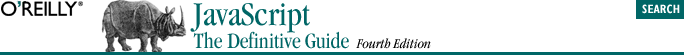home | O'Reilly's CD bookshelfs | FreeBSD | Linux | Cisco | Cisco Exam## 5.6. String Operators

`"hello" + " " + "there"`

And the following lines produce the string "22":

```a = "2"; b = "2";
c = a + b;```
```1 + 2        // Addition. Result is 3.
"1" + "2"    // Concatenation. Result is "12".
"1" + 2      // Concatenation; 2 is converted to "2". Result is "12".
11 < 3       // Numeric comparison. Result is false.
"11" < "3"   // String comparison. Result is true.
"11" < 3     // Numeric comparison; "11" converted to 11. Result is false.
"one" < 3    // Numeric comparison; "one" converted to NaN. Result is false.
// In JavaScript 1.1, this causes an error instead of NaN.```

Finally, it is important to note that when the + operator is used with strings and numbers, it may not be associative. That is, the result may depend on the order in which operations are performed. This can be seen with examples like these:

```s = 1 + 2 + " blind mice";   // Yields "3 blind mice"
t = "blind mice: " + 1 + 2;  // Yields "blind mice: 12"```

The reason for this surprising difference in behavior is that the + operator works from left to right, unless parentheses change this order. Thus, the last two examples are equivalent to these:

```s = (1 + 2) + "blind mice";    // 1st + yields number; 2nd yields string
t = ("blind mice: " + 1) + 2;  // Both operations yield strings```